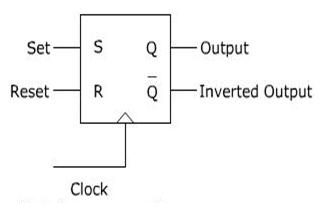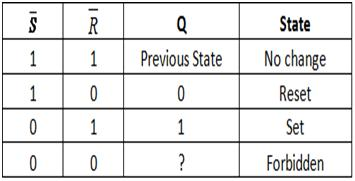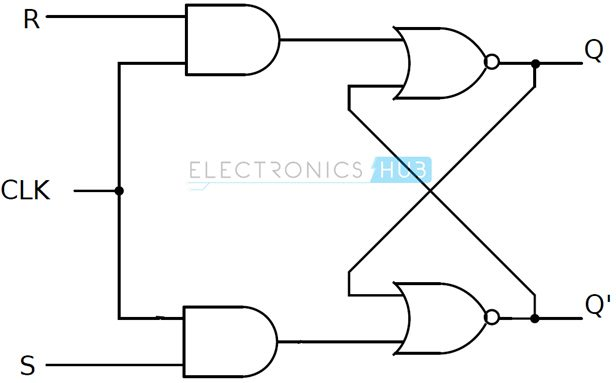## SR Flip Flop Design with NOR Gate and NAND Gate

### Introduction to SR Flip Flop

The SR flip – flop is one of the fundamental parts of the sequential circuit logic. SR flip – flop is a memory device and a binary data of 1 – bit can be stored in it. SR flip – flop has two stable states in which it can store data in the form of either binary zero or binary one. Like all flip – flops, an SR flip – flop is also an edge sensitive device.

SR flip – flop is one of the most vital components in digital logic and it is also the most basic sequential circuit that is possible. The S and R in SR flip – flop means ‘SET’ and ‘RESET’ respectively. Hence it is also called Set – Reset flip – flop. The symbolic representation of the SR Flip Flop is shown below.

#### Working

SR flip – flop works during the transition of clock pulse either from low – to – high or from high – to – low (depending on the design) i.e. it can be either positive edge triggered or negative edge triggered.

For a positive edge triggered SR flip – flop, suppose, if S input is at high level (logic 1) and R input is at low level (logic 0) during a low – to – high transition on clock pulse, then the SR flip – flop is said to be in SET state and the output of the SR flip – flop is SET to

1. For the same clock situation, if the R input is at high level (logic 1) and S input is at low level (logic 0), then the SR flip – flop is said to be in RESET state and the output of the SR flip – flop is RESET to 0.The SR flip – flops can be designed by using logic gates like NOR gates and NAND gates. Unclocked or simple SR flip – flops are same as SR Latches. The two types of unclocked SR flip – flops are discussed below

### Unclocked S-R Flip-Flop Using NAND Gate

SR flip flop can be designed by cross coupling of two NAND gates. It is an active low input SR flip – flop. The circuit of SR flip – flop using NAND gates is shown in below figure#### Working

`Case 1:`

When both the SET and RESET inputs are high, then the output remains in previous state i.e. it holds the previous data.

`Case 2:`

When SET input is HIGH and RESET input is LOW, then the flip flop will be in RESET state. Because the low input of NAND gate with R input drives the other NAND gate with 1, as its output is 1. So both the inputs of the NAND gate with S input are 1. This will cause the output of the flip – flop to settle in RESET state.

`Case 3:`

When SET input is LOW and RESET input is HIGH, then the flip flop will be in SET state. Because the low input of NAND gate with S input drives the other NAND gate with 1, as its output is 1. So both the inputs of the NAND gate with R input are 1. This will cause the output of the flip – flop to settle in SET state.

`Case 4:`

When both the SET and RESET inputs are low, then the flip flop will be in undefined state. Because the low inputs of S and R, violates the rule of flip – flop that the outputs should compliment to each other. So the flip flop is in undefined state (or forbidden state).

The table below summarizes above explained working of SR Flip Flop designed with the help of a NAND gates
(or forbidden state).### Unclocked S R Flip-Flop Using NOR Gate

SR flip flop can also be designed by cross coupling of two NOR gates. It is an active high input SR flip – flop. The circuit of SR flip – flop using NOR gates is shown in below figure.The operation is same as that of NOR SR Latch.

#### Working

Case 1:

When both the SET and RESET inputs are low, then the output remains in previous state i.e. it holds the previous data

`Case 2:`

When SET input is low and RESET input is high, then the flip flop will be in RESET state. Because the high input of NOR gate with R input drives the other NOR gate with 0, as its output is 0. So both the inputs of the NOR gate with S input are 0. This will cause the output of the flip – flop to settle in RESET state.

`Case 3:`

When SET input is high and RESET input is low, then the flip flop will be in SET state. Because the low input of NOR gate with S input drives the other NOR gate with 1, as its output is 1. So both the inputs of the NOR gate with R input are 1. This will cause the output of the flip flop to settle in SET state.

`Case 4:`

When both the SET and RESET inputs are high, then the flip flop will be undefined state. Because the high inputs of S and R, violates the rule of flip flop that the outputs should complement to each other. So the flip flop is in undefined state (or forbidden state).
The table below summarizes above explained working of SR Flip Flop designed with the help of a NOR gate.Even though simple SR flip – flops and simple SR latches are same, both the terms are used in their respective contexts.

The problem with simple SR flip – flops is that they are level sensitive to the control signal (although not shown in figure) which makes them a transparent device. In order to avoid this, Gated or Clocked SR flip – flops are introduced (whenever the term SR flip – flop is used, it usually refers to clocked SR flip – flop). Clock signal makes the device edge sensitive (and hence no transparency).

### Clocked SR Flip – Flops

Two types of clocked SR flip – flops are possible: based on NAND and based on NOR. The circuit of clocked SR flip – flop using NAND gates is shown belowThis circuit is formed by adding two NAND gates to NAND based SR flip – flop. The inputs are active high as the extra NAND gate inverts the inputs. A clock pulse is given as input to both the extra NAND gates.

Hence the transition of the clock pulse is a key factor in functioning if this device. Assuming it is a positive edge triggered device, the truth table for this flip – flop is shown below.The same can be achieved by using NOR gates. The circuit of clocked SR flip – flop using NOR gates is shown below.The figure suggests a structure of RS flip – flop (as R is associated to the output Q), the functionality of SET and RESET remain the same i.e. when S is high, Q is set to 1 and when R is high, Q is reset to 0.

### Applications

SR flip – flops are very simple but are not widely used in practical circuits because of their illegal state where both S and R are high (S = R = 1). But they are used in switching circuits as they provide simple switching function (between Set and Reset). One such application is a Switch de – bounce circuit. The SR flip – flops are used to eliminate mechanical bounce of switches in digital circuits.

#### Mechanical Bounce

Mechanical switches, when pressed or released, often take some time and vibrate several times before settling down. This non – ideal behavior of the switch is called switch bounce or mechanical bounce. This mechanical bounce will tend to fluctuate between low and high voltages which can be interpreted by digital circuit. This can result in variation of pulse signals and these series of unwanted pulses will result in the digital system to work incorrectly.For example, in this bouncing period of the signal, the fluctuations in the output voltage are very high and therefore the register counts several inputs instead of single input. To eliminate this kind of behavior of digital circuits, we use SR flip – flops.

#### How Does S R Flip-Flop Eliminates the Mechanical Bounce

Based on the present state output, if the set or reset buttons are depressed then the output will change in a manner that it counts more than one signal input i.e. the circuit may receive some unwanted pulse signals and thus because of the mechanical bouncing action of machines, there is no change in outputs at Q.

When the button is pressed, the contact will affect the flip flop input and there will be change in the present state and no further affects on the circuit/machine for any other mechanical switch bounces. If there is any additional input from the switch, there will be no change and SR flip – flop will reset after some small period of time.

So the same switch will come to use only after an SR flip – flop executes a state change i.e. only after receiving the single clock pulse signal.

The circuit of a switch de – bouncing circuit is shown below.The input to the switch is connected to ground (logic 0). There are two pull up resistors connected to each of the input. They ensure that flip – flop inputs S and R are always 1 when the switch is between contacts.
Another circuit can be constructed with NOR SR flip – flop.The input to the switch is connected to logic 1. There are two pull down resistors connected to each of the input. They ensure that flip – flop inputs S and R are always 0 when the switch is between the contacts a and b.

Commonly used ICs for eliminating the mechanical switch bounce are MAX6816 – single input, MAX6817 – dual input, MAX6818 – octal input switch de-bouncer ICs. These ICs contain the necessary configuration with the SR flip – flops.